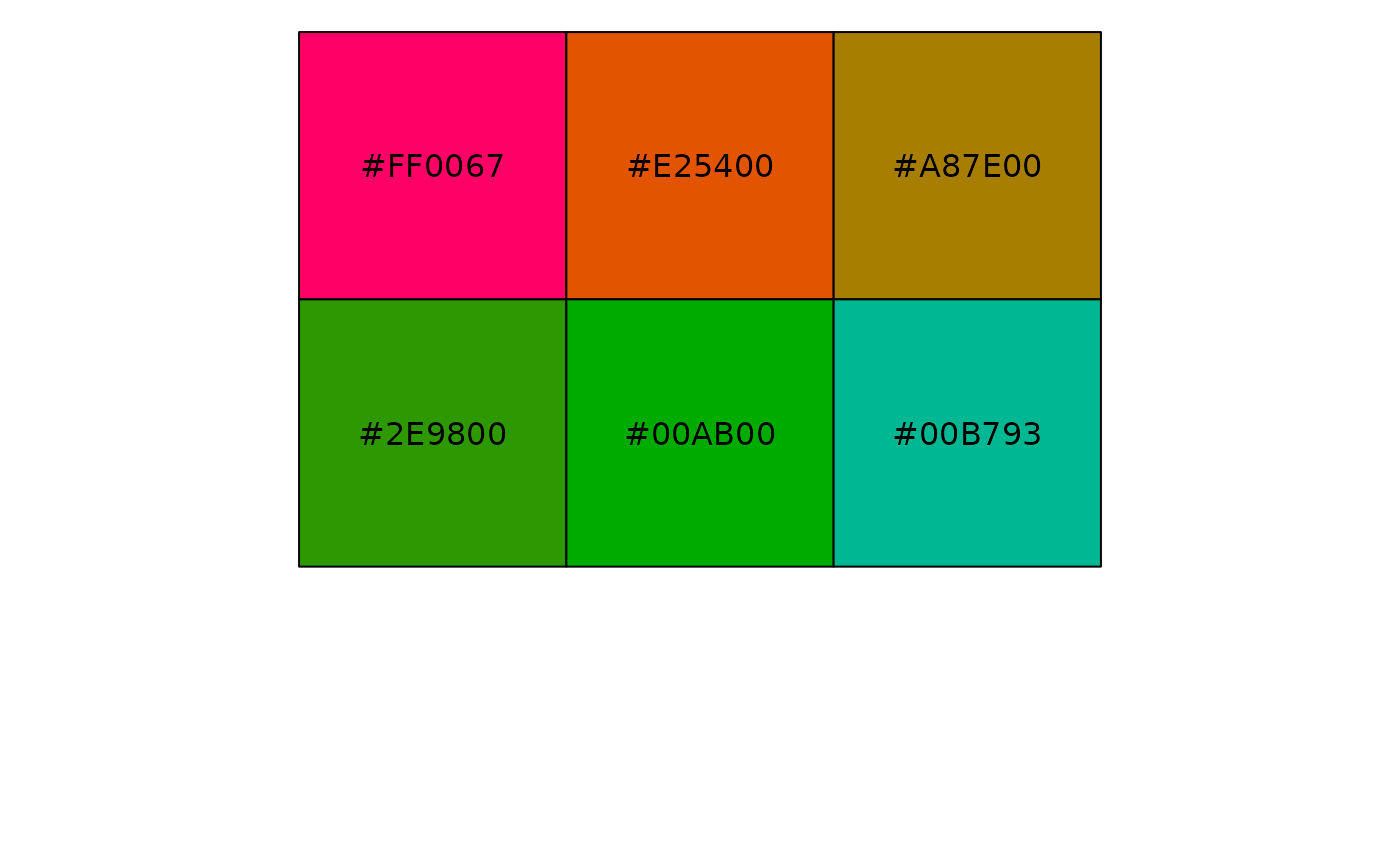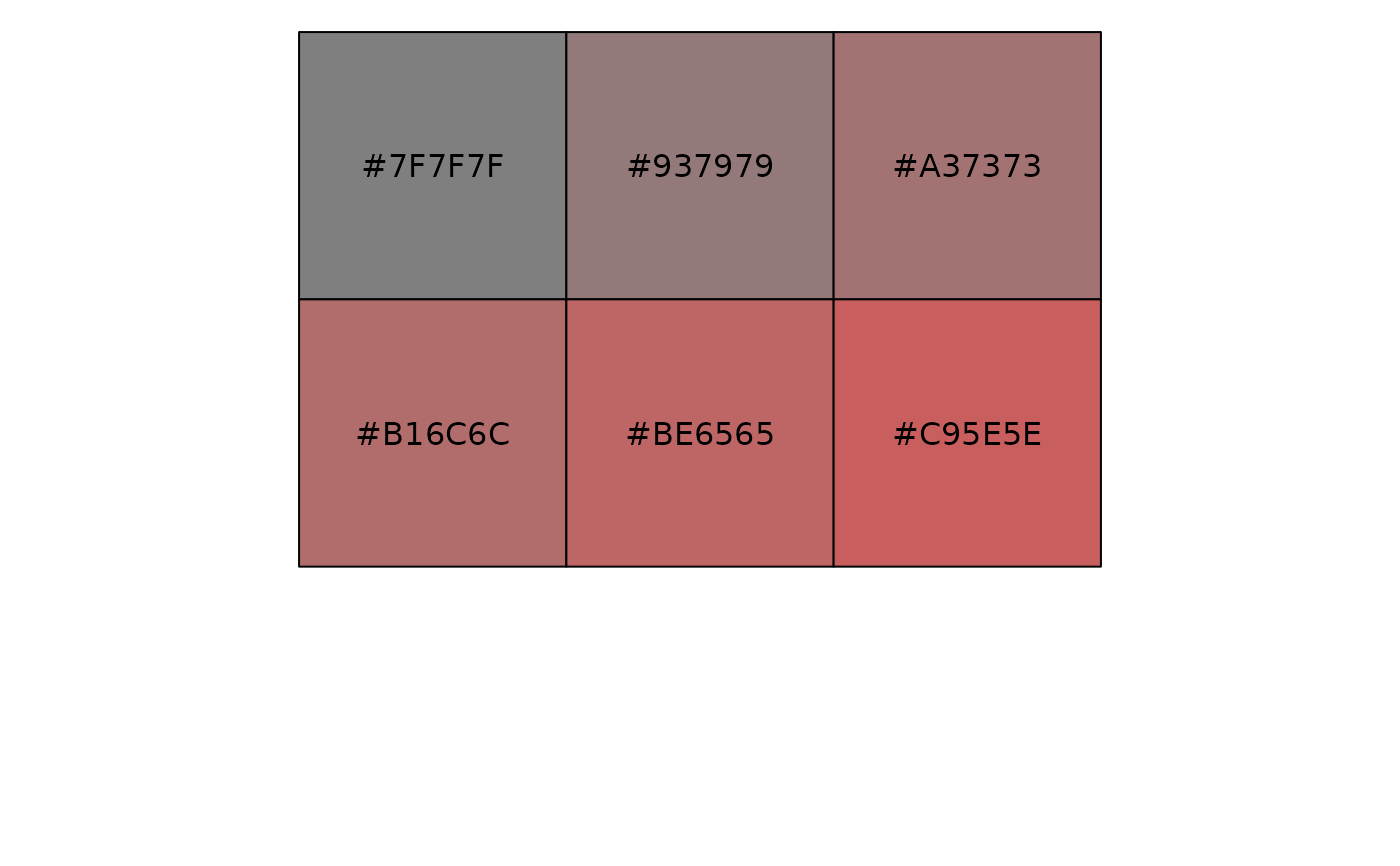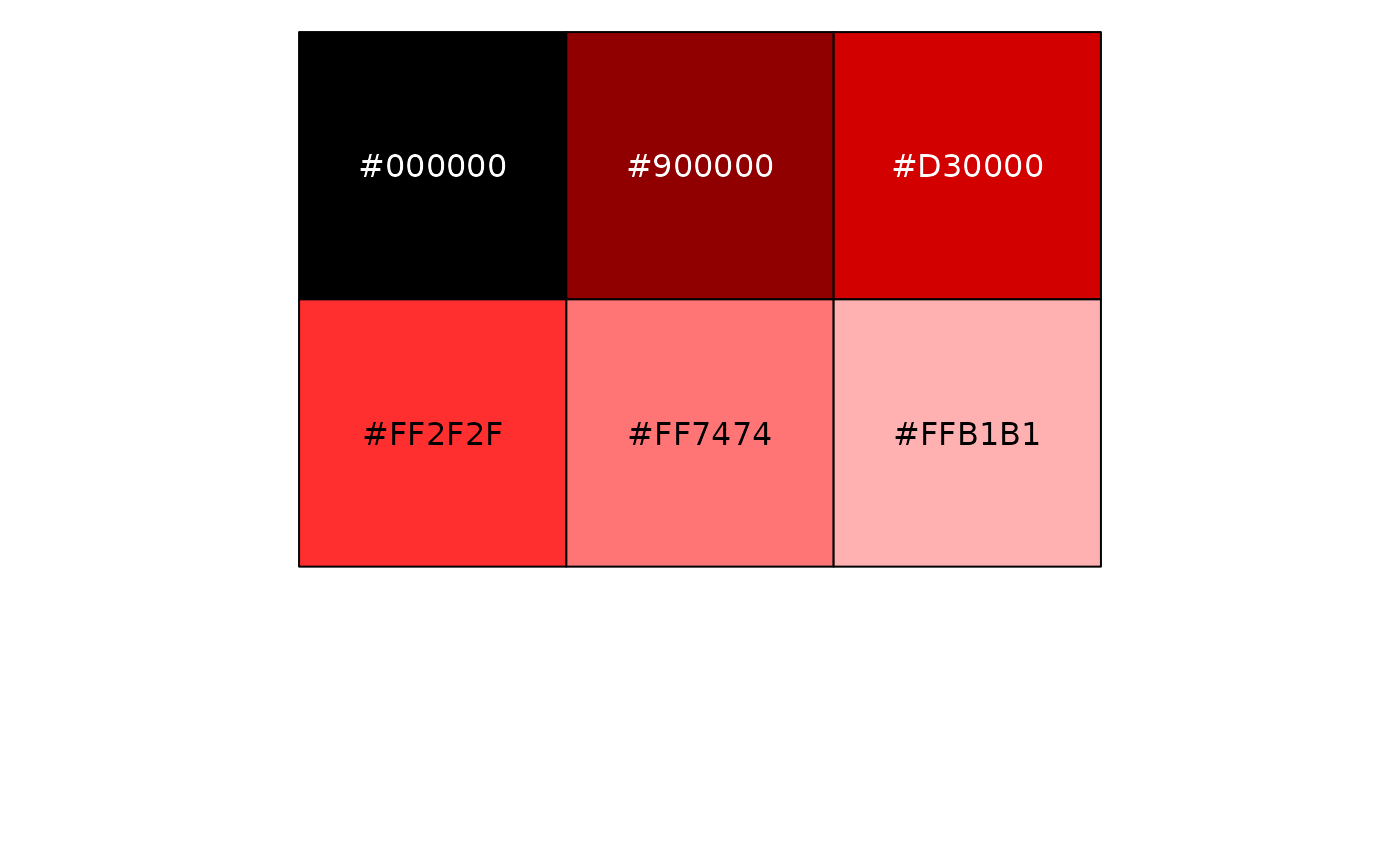Transforms rgb to hcl, sets non-missing arguments and then backtransforms to rgb.

## Usage

col2hcl(colour, h = NULL, c = NULL, l = NULL, alpha = NULL)

## Arguments

colour

character vector of colours to be modified

h

Hue, [0, 360]

c

Chroma, [0, 100]

l

Luminance, [0, 100]

alpha

Alpha, [0, 1].

## Examples

reds <- rep("red", 6)
show_col(col2hcl(reds, h = seq(0, 180, length = 6)))show_col(col2hcl(reds, c = seq(0, 80, length = 6)))show_col(col2hcl(reds, l = seq(0, 100, length = 6)))show_col(col2hcl(reds, alpha = seq(0, 1, length = 6)))